# Gas Laws Gas Pressure n Gas pressure is

• Slides: 26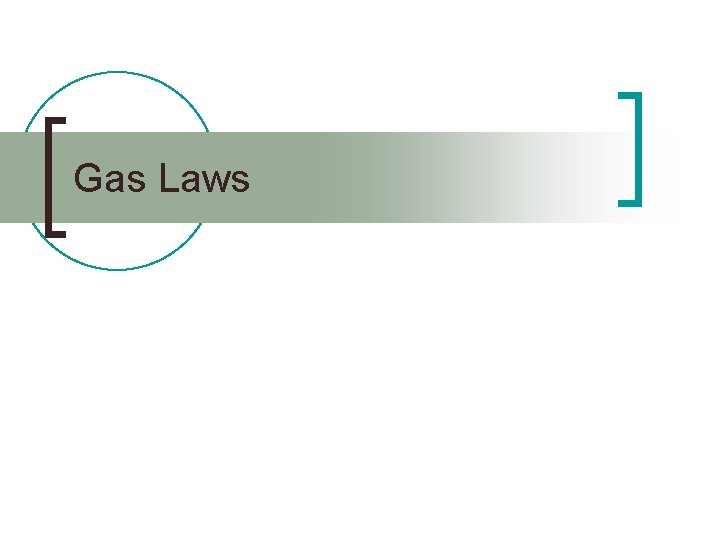Gas LawsGas Pressure n Gas pressure is the result of gas particles colliding with the walls of the container ¡ If the volume and temperature of the container is constant: n n If gas is added, pressure increases If gas is removed, pressure decreasesGas Pressure n Gas molecules move from an area of higher pressure to area of lower pressure ¡ Opening a can/bottle of soda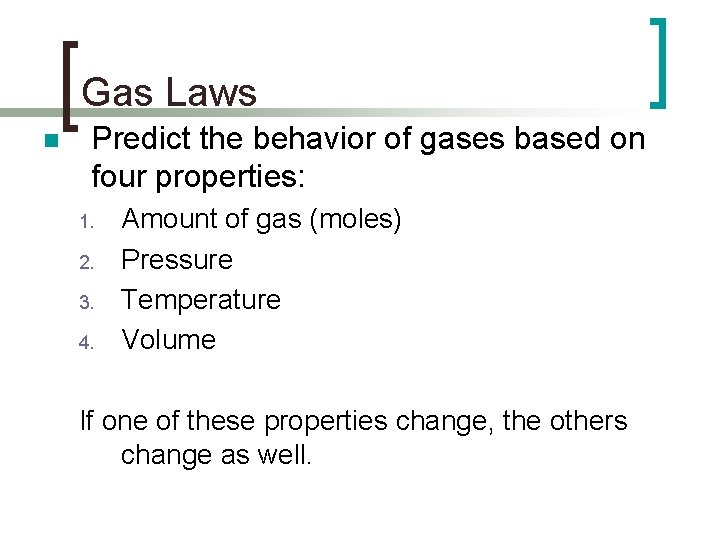Gas Laws n Predict the behavior of gases based on four properties: 1. 2. 3. 4. Amount of gas (moles) Pressure Temperature Volume If one of these properties change, the others change as well.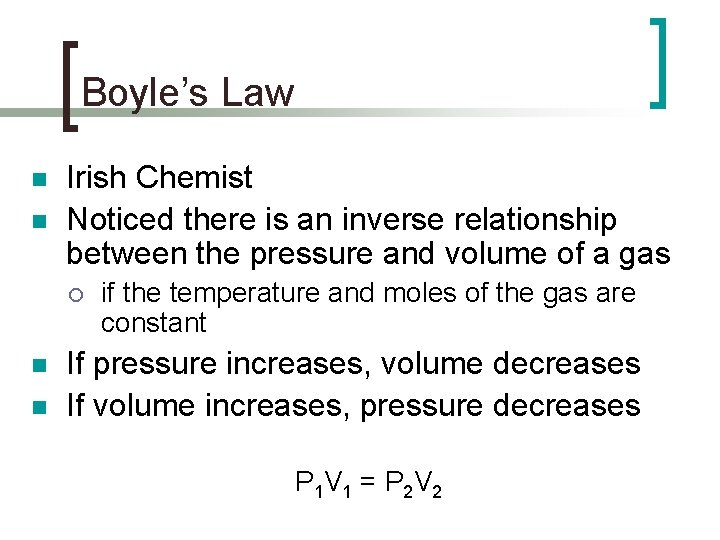Boyle’s Law n n Irish Chemist Noticed there is an inverse relationship between the pressure and volume of a gas ¡ n n if the temperature and moles of the gas are constant If pressure increases, volume decreases If volume increases, pressure decreases P 1 V 1 = P 2 V 2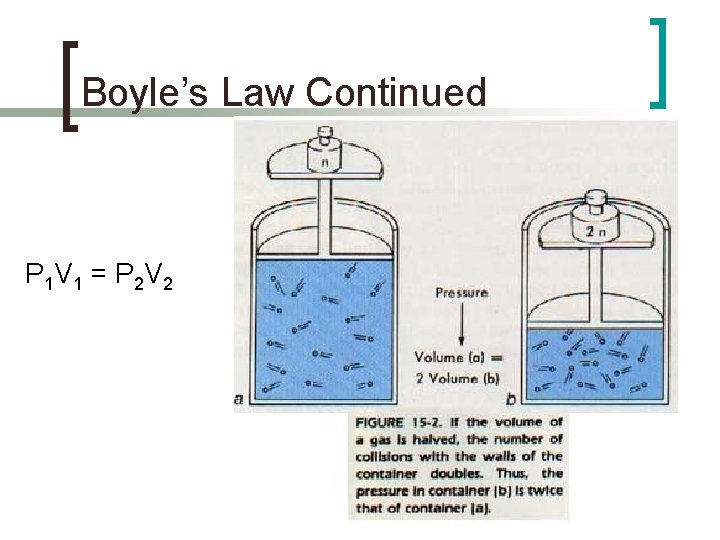Boyle’s Law Continued P 1 V 1 = P 2 V 2Practice Air in a container occupies 145. 7 m. L at 1. 08 atm. What volume must the container be to have a pressure of 1. 43 atm? 110. 04 m. L 1.Practice 1. A balloon filled with He has a volume of 4. 0 L has a pressure of 210 k. Pa. If the balloon is compressed to 2. 5 L, what will the pressure of He inside the balloon? Answer: 336 k. PaPractice 1. The volume of a gas at 99. 0 k. Pa is 300 m. L. If the pressure is increased to 188 k. Pa, what is the new volume? Answer: 157. 9 m. L 2. The pressure of a sample of He in a 1. 00 L container is 0. 988 atm. What is the new pressure if the sample is placed in a 2. 00 L container? Answer: 0. 494 atm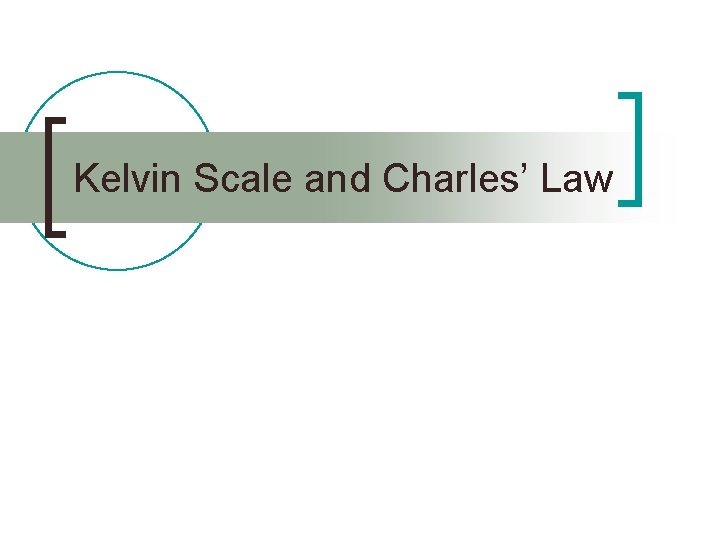Kelvin Scale and Charles’ LawTemperature Scales n n SI scale: degree Celsius (°C) or Kelvin (K) English scale: degree Fahrenheit (°F) n °C + 273 = Temperature in Kelvin – 273 = temperature in °C n Kelvin scale is based off the Celsius scale. n ¡ At 0 K all matter is believed to stop moving n Absolute Zero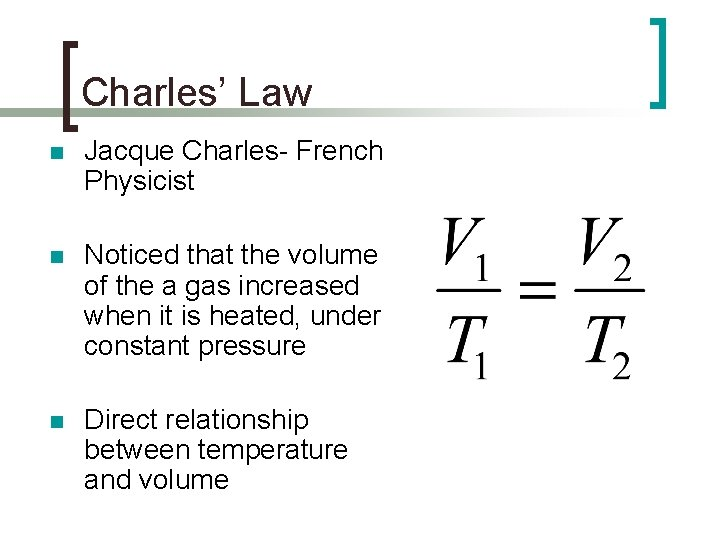Charles’ Law n Jacque Charles- French Physicist n Noticed that the volume of the a gas increased when it is heated, under constant pressure n Direct relationship between temperature and volume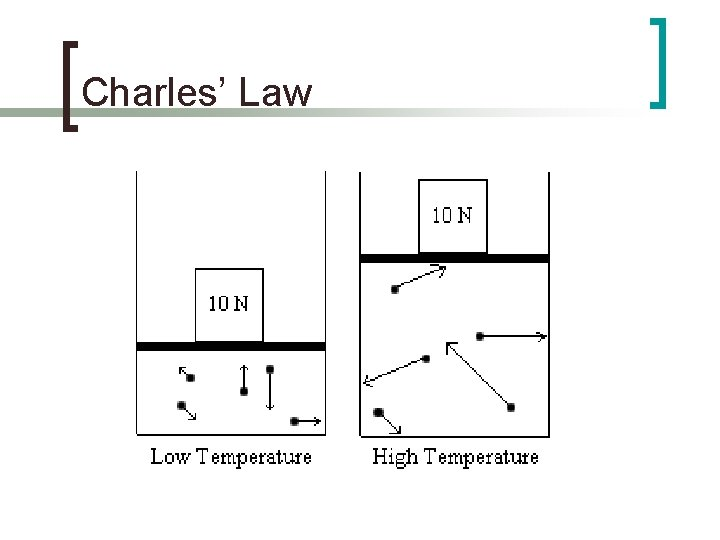Charles’ Law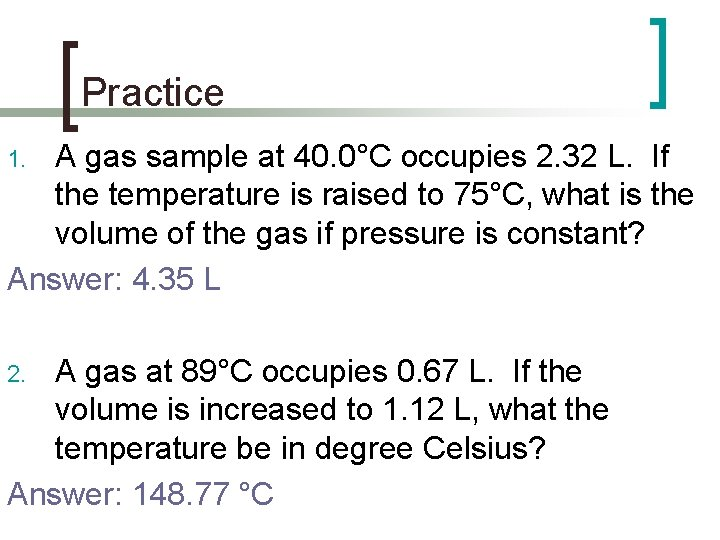Practice A gas sample at 40. 0°C occupies 2. 32 L. If the temperature is raised to 75°C, what is the volume of the gas if pressure is constant? Answer: 4. 35 L 1. A gas at 89°C occupies 0. 67 L. If the volume is increased to 1. 12 L, what the temperature be in degree Celsius? Answer: 148. 77 °C 2.Practice The Celsius temperature of a 300 L sample of gas is lowered from 80. 0 °C to 30. 0 °C. What will be the resulting volume of this gas? Answer: 112. 5 L 4. What is the volume of the air in a balloon that occupies 0. 620 L at 25 °C if the temperature is lowered to 0. 00 °C. (convert temps. to Kelvin) Answer: 0. 568 L 3.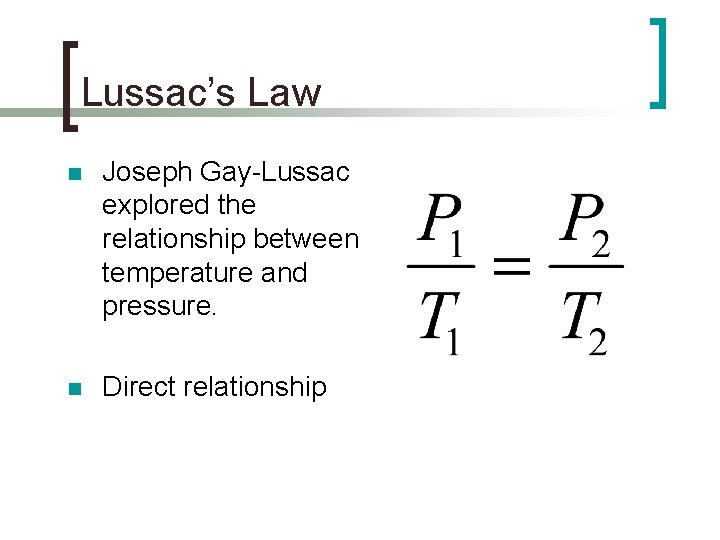Lussac’s Law n Joseph Gay-Lussac explored the relationship between temperature and pressure. n Direct relationshipPractice 1. A gas in a sealed container has a pressure of 123 k. Pa at 30. 0°C. If the pressure is increased to 201 k. Pa, what is the new temperature? Answer: 49. 02°C 2. The pressure in an automobile tire is 1. 88 atm at 25°C. What will be the pressure if the temperature warms to 37°C? Answer: 2. 78 atmCombined Gas Law n n Combines Boyle’s, Charles’s, and Lussac’s Laws. Shows the relationship between temperature, pressure, and volume if the amount of gas is held constant. P 1 V 1 = P 2 V 2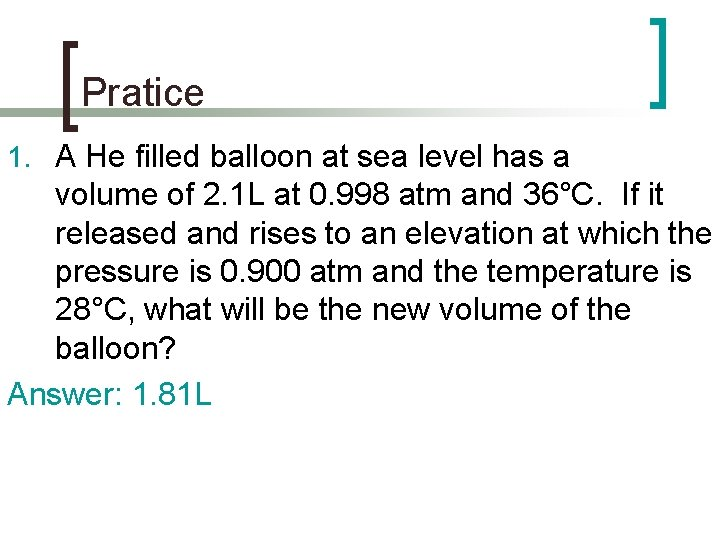Pratice 1. A He filled balloon at sea level has a volume of 2. 1 L at 0. 998 atm and 36°C. If it released and rises to an elevation at which the pressure is 0. 900 atm and the temperature is 28°C, what will be the new volume of the balloon? Answer: 1. 81 L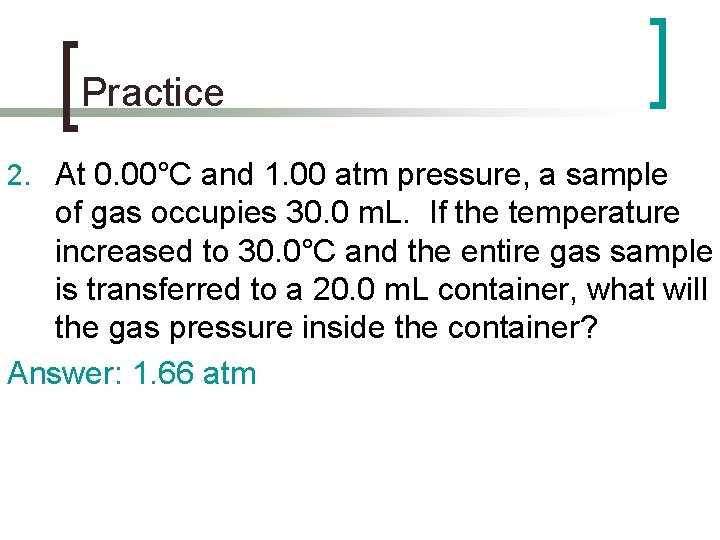Practice 2. At 0. 00°C and 1. 00 atm pressure, a sample of gas occupies 30. 0 m. L. If the temperature increased to 30. 0°C and the entire gas sample is transferred to a 20. 0 m. L container, what will the gas pressure inside the container? Answer: 1. 66 atmIdeal Gas Law n The properties of a gas under ideal conditions PV=n. RT P- pressure (atm or k. Pa) V- volume (L) n- moles of gas (moles) R- ideal gas constant T- temperature (K)What is the Ideal Gas Constant? n n n Determined by a series of experiments Relates pressure, volume, temperature, and moles. 2 Ideal Gas Constants ¡ ¡ n 0. 0821 8. 314 Which one do you use? ¡ Based on pressure units n n If pressure in atm use 0. 0821 If pressure in k. Pa use 8. 314Practice 1. Calculate the number of moles of a gas in a 3. 0 L vessel at 300. K with a pressure of 1. 50 atm.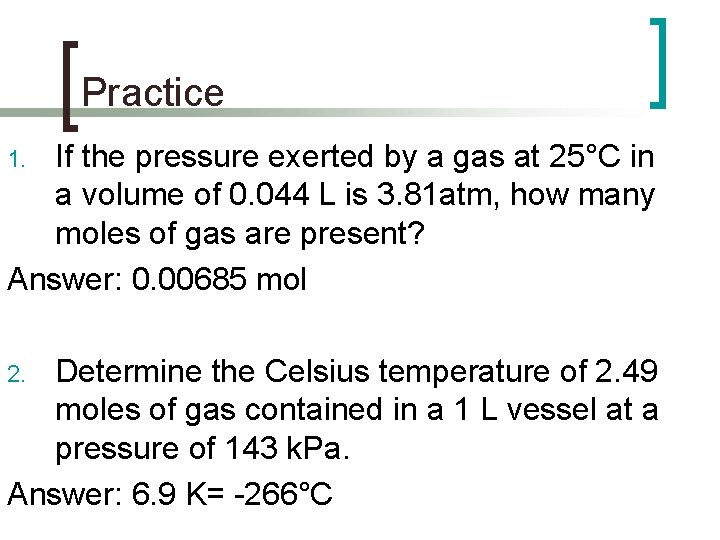Practice If the pressure exerted by a gas at 25°C in a volume of 0. 044 L is 3. 81 atm, how many moles of gas are present? Answer: 0. 00685 mol 1. Determine the Celsius temperature of 2. 49 moles of gas contained in a 1 L vessel at a pressure of 143 k. Pa. Answer: 6. 9 K= -266°C 2.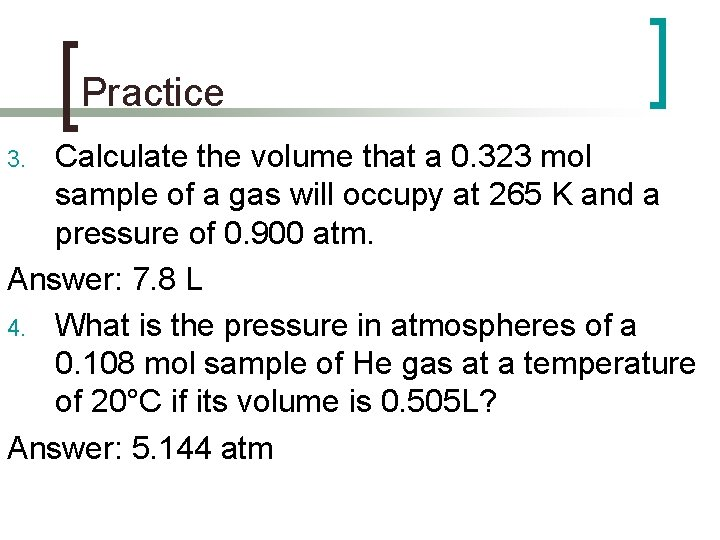Practice Calculate the volume that a 0. 323 mol sample of a gas will occupy at 265 K and a pressure of 0. 900 atm. Answer: 7. 8 L 4. What is the pressure in atmospheres of a 0. 108 mol sample of He gas at a temperature of 20°C if its volume is 0. 505 L? Answer: 5. 144 atm 3.Equations and Information PV=n. RT R= 0. 0821 R= 8. 314 R= 62. 4 P 1 V 1 = P 2 V 2 1 atm = 101. 3 k. Pa Convert All Temperatures To 1 atm = 760 mm. Hg Kelvin